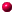"Inside  every small problem is a large problem struggling to get out."

RulesForumContributors[For contributors only]

## Thread and Full Text View

Respond to the question: A program to solve zero sum games?

 08/25/2012 08:41 AM by name withheld;

 04/04/2002 10:21 PM by David K. Levine; computing the value correctly
Thanks to Wayne Comeaux for pointing out that the zero-sum game solver wasn't computing the value correctly (it seemed always to give zero). I believe this to be fixed... [View full text and thread]

 03/26/2002 04:17 PM by David K. Levine; computing the value
OK, I think it will give the value of the game to the row player now. [View full text and thread]

I can't get it to work out the value of any games. [View full text and thread]

 02/12/2000 02:04 PM by David K. Levine; Calculating the value
The zero sum game solver now calculates the value of the game as well as the optimal player 2 strategy. [View full text and thread]

 01/15/2000 10:03 AM by David K. Levine; There is a program to solve zero sum games

There is a program to solve zero sum games on this site at http://levine.sscnet.ucla.edu/Games/zerosum.htm. This program uses the fact that finding the value of a zero sum game is equivalent to solving a linear programming problem. Unfortunately, I cannot release the source code for the program because it solves linear programs using the simplex method, and the source code for the simplex method is taken from Numerical Recipes. Their license agreement does not allow me to redistribute this source code.

The zerosum program does not provide all the information you want because it provides the strategies for the second player only, and it does not compute the value. However, you can easily get the remaining information you need from the program. For example, consider payoffs to the first player of

 1 2 3 -4

Using the solver, we find the optimal player 2 strategy is .75, .25. These are the probabilities of columns. To find the value, calculate the payoff to rows when these are the column probabilities. The first row gives 1*.75+2*.25=1.25; the second row gives 3*.75-4*.25=1.25. The fact that both rows give the same payoff is typical of optimal player two strategies. The value of the game (to player 1) is the largest of these row payoffs, in this case 1.25.

To find the optimal player 1 strategies, we simply reverse the role of the players, plugging in the payoff matrix with rows and column interchanged and the negative of the original payoffs

 -1 -3 -2 4

From this we find the optimal strategy for the original player 1 of .875, .125.

Your question asked not just about programs to find optimal strategies and the value, but also about a particular method of solution, called iterated play. This method, more commonly known as fictitious play, has each player start with an arbitrary play in the first period. In each subsequent period, the player believes that his opponent will play the average frequency used in past periods, and plays a best response to it. In zero-sum games this method converges to the optimal strategies. It need not for non-zero sum games, as shown by an example of Shapley [Shapley, L. : "Some Topics in Two-Person Games," Advances in Game Theory, M. Drescher, L.S. Shapley, and A.W. Tucker, Princeton University Press]. Convergence in the zero sum case was first shown by Brown [Brown, G. W. : "Iterative Solutions of Games by Fictitious Play," Activity Analysis of Production and Allocation, T.C. Koopmans,  Wiley].

To the best of my knowledge no one has implemented the fictitious play method on the computer to solve zero sum games. The reason for this is that the iterative method can be quite slow, so the simplex method is preferred.

[Manage messages]

 01/15/2000 08:37 AM by name withheld; A program to solve zero sum games?
Is there a program that searches for saddle points and gives the solution of a two person zero sum game, including its value as well as the player strategies? I remember learning as a student a method of solving through iterated play. [View full text and thread]